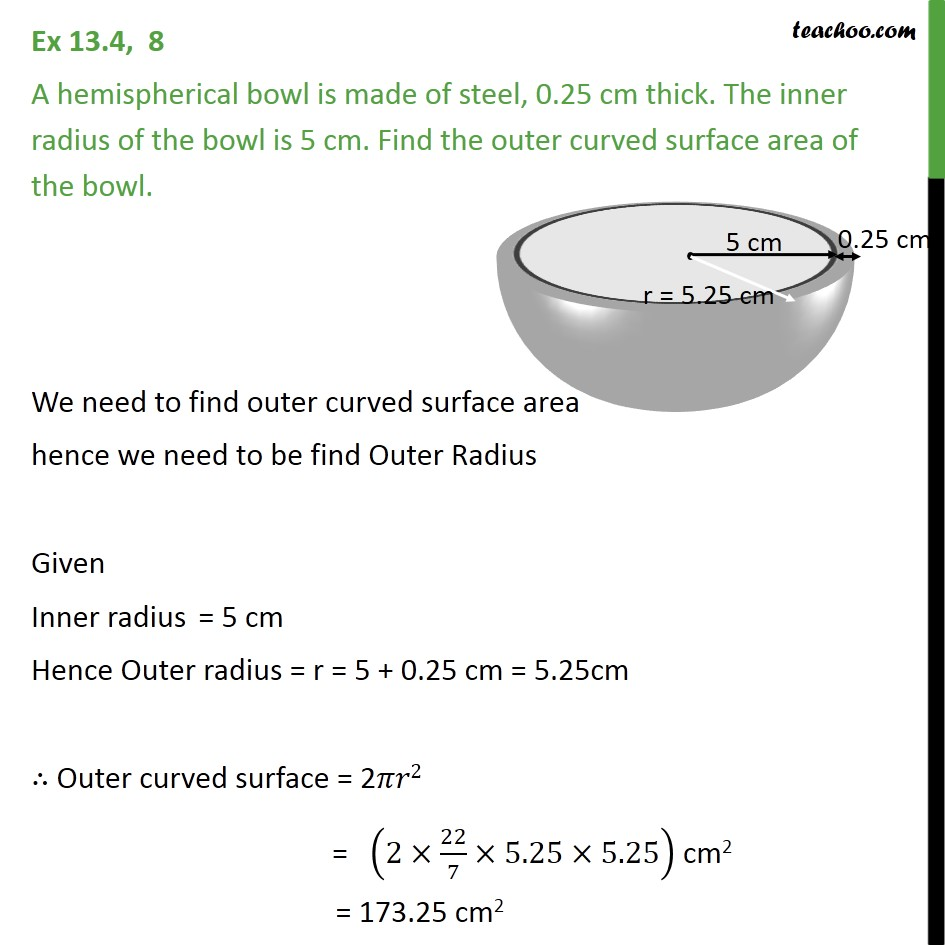1. Chapter 13 Class 9 Surface Areas and Volumes (Term 2)
2. Serial order wise
3. Ex 13.4

Transcript

Ex 13.4, 8 A hemispherical bowl is made of steel, 0.25 cm thick. The inner radius of the bowl is 5 cm. Find the outer curved surface area of the bowl. We need to find outer curved surface area hence we need to be find Outer Radius Given Inner radius = 5 cm Hence Outer radius = r = 5 + 0.25 cm = 5.25cm Outer curved surface = 2 2 = (2 22/7 5.25 5.25) cm2 = 173.25 cm2